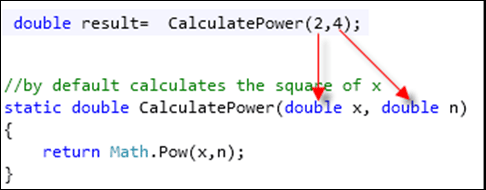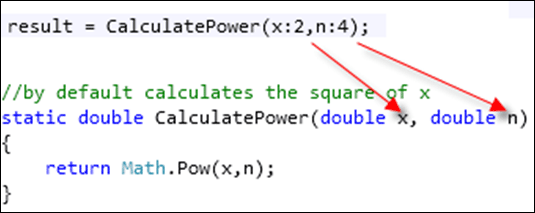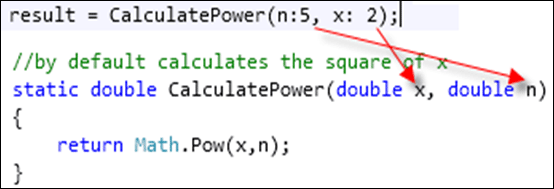# Named arguments in C# 4.0

This new feature of c# 4.0 allows users to define arguments in the calling statement in any order rather than defined in the parameter list (Argument list references the calling statement and parameter list references the method prototype).  With this new feature the user can call a method by passing arguments irrespective of their actual position. Thus the users are free from the headache of remembering the position of the parameters while calling a method:

//calculates the n to the power of x
static double CalculatePower(double x, double n)
{
return Math.Pow(x,n);
}

User calls this method in following:
1. Following image depicts the calling way and the order of the assignment of the values to the parameter2. Following image depicts the second calling way and the order of the assignment of the values to the parameter3. Following image depicts the third calling way and the order of the assignment of the values to the parameterFollowing is the output:Let us elaborate the output

When user calls the function using following line the x will get 2 as its value and n will get 4 as its value

double result=  CalculatePower(2,4);

and following is the result printed because of 24

Result is :16

When user calls the function using following line the x will get 2 as its value and n will get 4 as its value

result = CalculatePower(x:2,n:4);

and following is the result printed because of 24

Result is :16

When user calls the function using following line the x will get 5 as its value and n will get 2 as its value

result = CalculatePower(5,2);

and following is the result printed because of 52

Result is :25

When user calls the function using following line the x will get 2 as its value and n will get 5 as its value

result = CalculatePower(n:5, x: 2);

and following is the result printed because of 25

Result is :32

erver'>